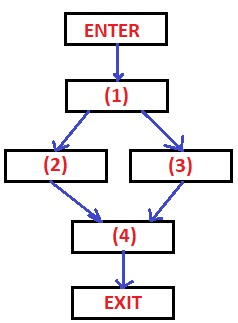### Loops in flow graphs

LOOPS IN FLOW GRAPHS:

Take source code as shown below.
a = 0;
b = b+c;
c = 0;
If (a>d)
{
c = b;
b++;
}
else
{
c = d;
d++;
}
a= b+d;

Basic blocks in above source code are-
 (1) (2) (3) (4) a = 0; b = b+c; c = 0; If (a>d) c = b; b++; c = d; d++; a= b+d;

Flow graph for above Basic blocks -